# Algebra 1 : How to find slope of a line

## Example Questions

← Previous 1 3 4 5 6 7 8 9 17 18

### Example Question #1 : How To Find Slope Of A Line

What is the slope of the equation 4x + 3y = 7?

–7/3

3/4

–3/4

4/3

–4/3

–4/3

Explanation:

We should put this equation in the form of y = mx + b, where m is the slope.

Isolate the y term: 3y = 7 – 4x

Divide by 3: y = 7/3 – 4/3 * x

Rearrange terms: y = –4/3 * x + 7/3, so the slope is –4/3.

### Example Question #2 : How To Find Slope Of A Line

Find the slope of the line through the points (6,2) and (3,4).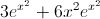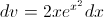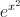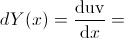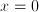Explanation:

The equation for slope is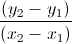. You plug in the coordinates from the points given you, and get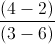, giving you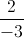. Note that it does not matter which point you use as point 1 and point 2, as long as you are consistent.(6,2) = (x1,y1)

(3,4) = (x2,y2)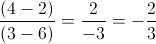### Example Question #1 : How To Find Slope Of A Line

Given the line 4y = 2x + 1, what is the slope of this line?

1/2

1/4

2

–1/4

–2

1/2

Explanation:

4y = 2x + 1 becomes y = 0.5x + 0.25. We can read the coefficient of x, which is the slope of the line.

4y = 2x + 1

(4y)/4 = (2x)/4 + (1)/4

y = 0.5x + 0.25

y = mx + b, where the slope is equal to m.

The coefficient is 0.5, so the slope is 1/2.

### Example Question #1 : How To Find Slope Of A Line

What is the slope of the line containing the points (7,12) and (91,32).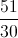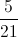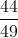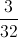Explanation:

To find the slope of a line you must first assign variables to each point. It does not matter which points get which variables as long as you keep the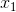and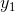and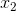and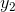consistent when you plug them into the equation.

Then we plug in the variables to this equation whererepresents the slope.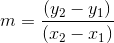Then we plug in our points for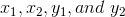and the example looks like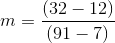Then we perform the necessary subtraction and division to find an answer of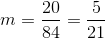### Example Question #5 : How To Find Slope Of A Line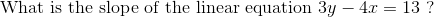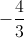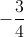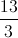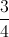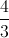Explanation: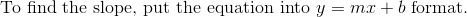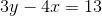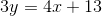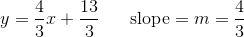### Example Question #6 : How To Find Slope Of A Line

Which of the following is an example of an equation written in slope-intercept form?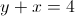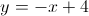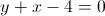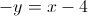Explanation:

Slope intercept form is, whereis the slope andis the y-intercept.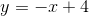is the correct answer. The line has a slope ofand a y-intercept equal to.

### Example Question #1 : How To Find Slope Of A Line

If (1,2) and (4,6) are on the same line, what is the slope of the line?Explanation: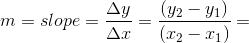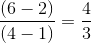### Example Question #3 : How To Find Slope Of A Line

The equation of a line is: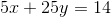What is the slope of the line?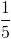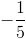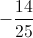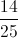Explanation:

Solve the equation forwhereis the slope of the line: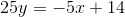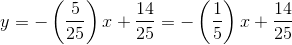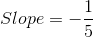### Example Question #1 : How To Find Slope Of A Line

A line passes through the points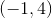and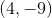.  What is its slope?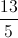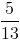-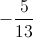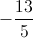Explanation:

The slope is the rise over the run.  The line drops in-coordinates by 13 while gaining 5 in the-coordinates.

### Example Question #10 : How To Find Slope Of A Line

What is the slope of the line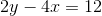?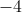You can rearrangeto get an equation resembling theformula by isolating the. This gives you the equation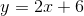. The slope of the equation is 2 (thewithin theequation).